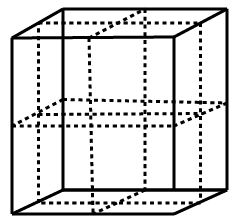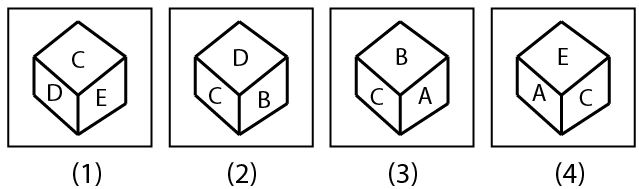# SSC CGL 2013 Tier 1 21 April Morning Shift

Instructions

In the following questions, from the given alternatives select the word which cannot be formed using letters of the given word.

Question 181

If - stands for addition, ÷ for multiplication, × for substraction, and + for division, then which of the following is correct ?

Question 182

A direction pole was situated on the Road Crossing. Due to an accident, the pole turned in such a manner that the pointer which was showing East, started showing South. Sita, a traveller went to the wrong direction thinking it to be West. In what direction actually she was travelling ?

Question 183

Ram cycled 10 km southward from his home, turned right and cycled 6 km, turned right, cycled 10 km, turned left and cycled 15 km. How many km will he have cycled to reach straight home ?

Question 184

Which of the following interchange of signs would make the given equation correct ?
5 + 3 × 8 - 12 ÷ 4 = 3

Question 185

Which of the following interchange of sign would make the given equation correct ?
(20 - 4) × 4 + 16 = 36

Question 186

If WATER is written as YCVGT, then what is written as HKTG ?

Instructions

In the following questions, two statements are given followed by two conclusions I and II. You have to consider the two statements to be true even if they seem to be at variance from commonly known facts. You have to decide which of the given conclusions, if any, follow from the given statements.

Question 187

Statements :
I. Some keys are locks, some locks are numbers.
II. All numbers are letters, all letters are words.
Conclusions :
I. Some words are numbers.
II. Some locks are letters.

Question 188

Statements :
I. The constitution assures the fundamental rights.
II. Parliament has right to amend the constitution.
Conclusions :
I. Parliament included fundamental rights in the constitution.
II. Parliament did not assure the fundamental rights.

Instructions

For the following questions answer them individually

Question 189

A cube which is painted red on the outer surface is of 2 inches height, 2 inches wide and 2 inches across. If it is cut into one-inch cubes as shown by dotted lines, indicate the number of cubes which are red on two sides only ?Question 190

Four positions of dice are given below. Which letter will be opposite to D ?OR